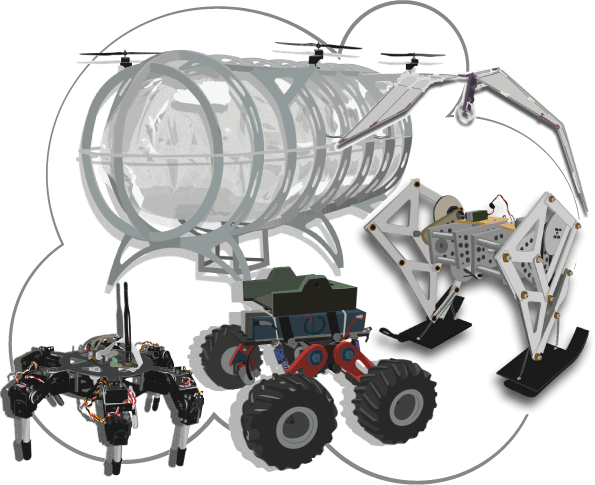# IntroductionThe Robotics Laboratory carries out basic and applied research activities in the field of computational mobile robotics spanning: robotic structures design, modeling physical systems, simulation and applied control of actuated, under-actuated and bionic robotics. One of the approaches of major interest of our group is on mechanical design, mathematical analysis and numerical modeling of physical robotic systems in the four modalities: rolling, walking, swimming and flying robots.

The Robotics Laboratory is organized by the research group of Mechatronics and was founded in August 2007 by Dr. Edgar A. Martínez-García. Our premise in the Robotics Laboratory is to promote theoretical analysis, applied mathematical techniques and computational simulation of robotics, as well as experimental implementation.

Our research approach is oriented on the development of individual student's projects, as a common strategy to achieve effective skills developments. In the laboratory we prepare students in multidisciplinary engineering activities, for them to be able to develop theoretical and practical skills in robotics.

The aim on research is to develop new models of autonomous robots. Some particular pursuits of our research group are:

1. Mathematical modeling and simulation of physical robotic systems.
2. Applied dynamic control of mobile robotic functions: navigation, planning and sensing.
3. Design, analysis and modeling of under-actuated robotic mechanisms.
4. Analysis and modeling of bionic robots: biomechanic models and their motion.
5. Research on deterministic sensor fusion models by physics-based integro-differential equations.
6. Scientific computing and mathematical methods for robot modeling.